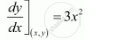Share

# Find the Points on the Curve Y = X3 at Which the Slope of the Tangent is Equal to the Y-coordinate of the Point. - CBSE (Commerce) Class 12 - Mathematics

#### Question

Find the points on the curve y = x3 at which the slope of the tangent is equal to the y-coordinate of the point.

#### Solution

The equation of the given curve is y = x3.

:. dy/dx = 3x^2

The slope of the tangent at the point (xy) is given by,When the slope of the tangent is equal to the y-coordinate of the point, then y = 3x2.

Also, we have y = x3.

∴3x2 = x3

⇒ x2 (x − 3) = 0

⇒ x = 0, x = 3

When x = 0, then y = 0 and when x = 3, then y = 3(3)2 = 27.

Hence, the required points are (0, 0) and (3, 27).

Is there an error in this question or solution?

#### APPEARS IN

NCERT Solution for Mathematics Textbook for Class 12 (2018 to Current)
Chapter 6: Application of Derivatives
Q: 17 | Page no. 212

#### Video TutorialsVIEW ALL 

Solution Find the Points on the Curve Y = X3 at Which the Slope of the Tangent is Equal to the Y-coordinate of the Point. Concept: Tangents and Normals.
S